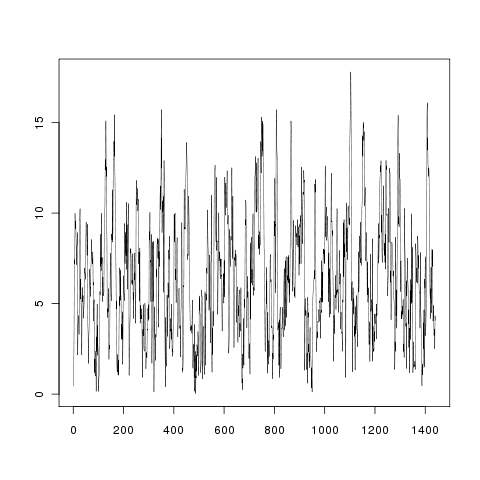Want to share your content on R-bloggers? click here if you have a blog, or here if you don't.

If you need to generate synthetic wind speed time series, you may find useful the procedure described in  “A Markov method for simulating non-gaussian wind speed time series” by G.M. McNerney and P.S. Veers (Sandia Laboratories, 1985), and “Estimation of extreme wind speeds with very long return periods” by M.D.G Dukes and J.P. Palutikof (Journal of applied meteorology, 1994). This procedure is internally implemented in the software Hybrid2 and Homer. I have implemented this procedure with R. The script is available here and is described below:

First, we have to define the parameters of the wind speed distribution:

```MeanSpeed<-6
MaxSpeed<-30
nStates<-30;

nRows<-nStates;
nColumns<-nStates;

##Position of each state
LCateg<-MaxSpeed/nStates;

##A Rayleigh fdp is a weibull with shape factor 2
Shape=2;
##and scale factor sigma*sqrt(2),
Scale=2*MeanSpeed/sqrt(pi);
```

Now, we build a vector of  wind speeds centered around the mean value of each state and obtain the density function defined by Shape and Scale:

```##Vector of wind speeds
WindSpeed=seq(LCateg/2, MaxSpeed-LCateg/2, by=LCateg);
##FDP of wind speeds (P matrix)
fdpWind<-dweibull(WindSpeed,shape=Shape, scale=Scale);
fdpWind<-fdpWind/sum(fdpWind);
```

The correlation between the different categories of wind speed is defined by a matrix constructed with a decreasing function:

```##Decreasing function
g<-function(x){2^(-abs(x))}

G<-matrix(nrow=nRows,ncol=nColumns)
G <- row(G)-col(G)
G <- g(G)
```

Then, an iterative procedure is needed for the calculation of the matrix of initial probability (P matrix):

```##Initial value of the P matrix
P0<-diag(fdpWind);
##Initial value of the p vector
p0<-fdpWind;

#The iterative procedure should stop when reaching a predefined error. However, for simplicity I have only constructed a for loop. To be improved!
steps=10;
P=P0;
p=p0;
for (i in 1:steps){
r<-P%*%G%*%p;
r<-as.vector(r/sum(r))
p=p+0.5*(p0-r)#vector
P=diag(p)}#matrix
```

Let’s combine the p vector and P and G matrices for obtaining a Markov Transition Matrix:

```N=diag(1/as.vector(p%*%G));
MTM=N%*%G%*%P
MTMcum<-t(apply(MTM,1,cumsum));
```

With this cumulated MTM we are able to simulate a wind speed time series:

```##One value per minute for 1 day
LSerie<-24*60;
#Random number for choosing the state
RandNum1<-runif(LSerie);
State<-InitialState<-1;
StatesSeries=InitialState;
##The next iterative procedure chooses the next state whose random number is greater than the cumulated probability defined by the MTM
for (i in 2:LSerie) {
State=min(which(RandNum1[i]<=MTMcum[State,]));
StatesSeries=c(StatesSeries,State)}

##Another random number to choose wind speeds inside each category
RandNum2<-runif(LSerie);
##And the wind speed series is
SpeedSeries=WindSpeed[StatesSeries]-0.5+RandNum2*LCateg;
##where the 0.5 correction is needed since the the WindSpeed vector is centered around the mean value of each category.
```

A result of this procedure is displayed in the next figure:We can check that the distribution of this wind speed series is correct:

```library(MASS)
print(fitdistr(SpeedSeries, 'weibull'))
```